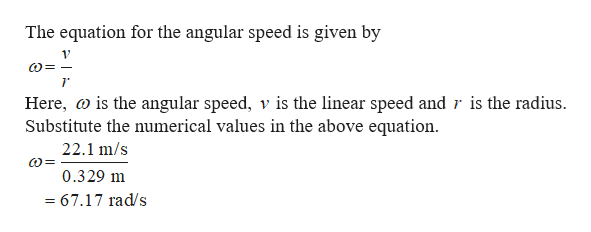# An automobile tire has a radius of 0.329 m, and its center moves forward with a linear speed of v = 22.1 m/s. (a) Determine the angular speed of the wheel. (b) Relative to the axle, what is the tangential speed of a point located 0.159 m from the axle?

Question
1 views

An automobile tire has a radius of 0.329 m, and its center moves forward with a linear speed of v = 22.1 m/s. (a) Determine the angular speed of the wheel. (b) Relative to the axle, what is the tangential speed of a point located 0.159 m from the axle?

check_circle

Step 1help_outlineImage TranscriptioncloseThe equation for the angular speed is given by Here, is the angular speed, v is the linear speed and r is the radius Substitute the numerical values in the above equation 22.1 m/s 0.329 m =67.17 rad/s fullscreen

### Want to see the full answer?

See Solution

#### Want to see this answer and more?

Solutions are written by subject experts who are available 24/7. Questions are typically answered within 1 hour.*

See Solution
*Response times may vary by subject and question.
Tagged in

### Angular Motion